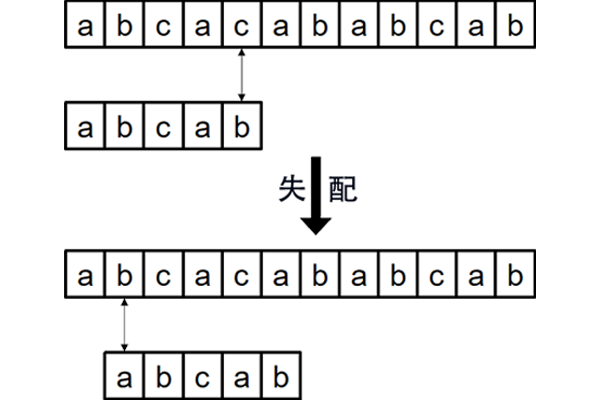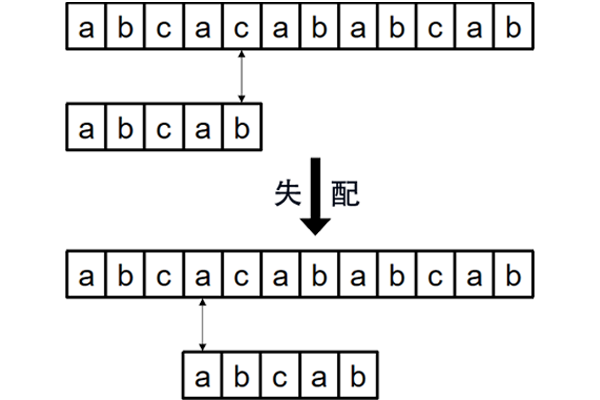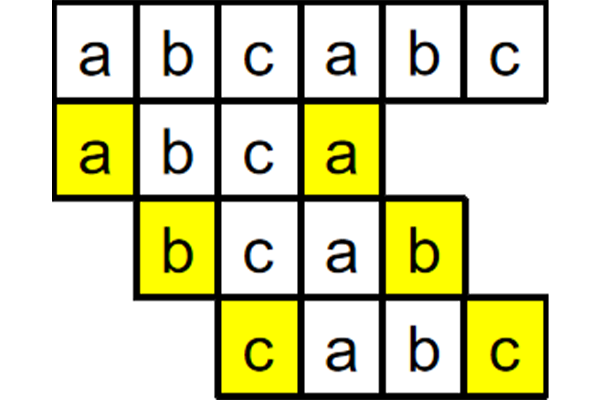# 什么是 $\mathbf{KMP}$

$KMP$ 算法是一种改进的字符串匹配算法，由$D.E.$ K $nuth$，$J.H.$ M $orris$和$V.R.$ P $ratt$提出的，因此人们称它为克努特—莫里斯—普拉特操作（简称 $KMP$ 算法）。 $KMP$ 算法的关键是利用匹配失败后的信息，尽量减少模式串与主串的匹配次数以达到快速匹配的目的。具体实现就是实现一个 $next()$ 函数，函数本身包含了模式串的局部匹配信息。时间复杂度 $\Theta(m+n)$ 。

（From 百度百科）

# $\mathbf{KMP}$ 算法的思想# 结语

$KMP$ 算法具有一种“最优历史处理”的性质，而这种性质也是基于 $KMP$ 的核心思想的。考虑前缀与后缀的关系使得其在匹配的时候不会反反复复地找。最后放上模板和 $std$ : P3375 【模板】KMP字符串匹配